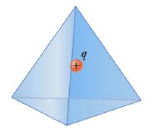Chapter 15, Problem 48P

Chapter
Section
Textbook Problem

A charge q = +5.80 μC is located at the center of a regular tetrahedron (a four-sided surface) as in Figure P15.48. Find (a) the total electric flux through the tetrahedron and (b) the electric flux through one face of the tetrahedron.Figure P15.48

(a)

To determine
The total electric flux.

Explanation

Given info: The charge q is 5.80μC .

Formula to calculate the electric flux is,

ϕE=qinsideε0

• qinside is the charge inside the surface.
• ε0 is the permittivity of free space.

Substitute 8.854×1012 m3kg1s4A2 for ε0 , 5.80μC for qinside

ϕE=5

(b)

To determine
The electric flux through one face of the tetrahedron.

Still sussing out bartleby?

Check out a sample textbook solution.

See a sample solution

The Solution to Your Study Problems

Bartleby provides explanations to thousands of textbook problems written by our experts, many with advanced degrees!

Get Started